cancel
Showing results for
Did you mean:Frequent Visitor

## Show multiple grand totals - row & column

Hello,

I am working on a dashboard in which I have a Matrix which has several measures and the totals are manipulated and fixed using the following approach:
if(COUNTROWS(values('Customer'[Country]))=1, [Measure_Impact], CALCULATE(sum ('Customer'[Impact])- [Measure_Impact_Correction]))

If I sum the total column values and the toal row values they do not match and it is ok as they are considering different metrics. What I can see in the bottom right corner of the Matrix is the sum of the total row values.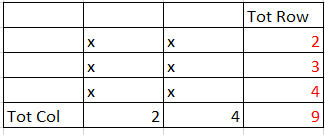I'd like to show two different values in this cell; i.e., the sum of the values of the last row and the sum of the values of the last column. Is it possible?  (Like in the picture below)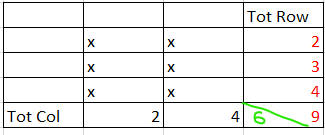Community Support

Hi @artur4 ,

Unfortunately, as far as I know so far, you can't display two values in a matrix cell at the same time, it shows the value you calculated.

I can take a simple test: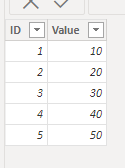Then I create the measures:

``Average = CALCULATE(AVERAGE('Table'[Value]), ALLEXCEPT('Table','Table'[ID]))``
``Sum = CALCULATE(SUM('Table'[Value]), ALLEXCEPT('Table', 'Table'[ID]))``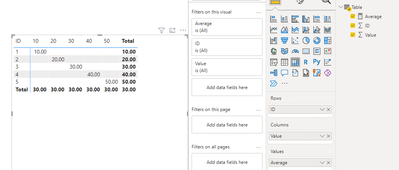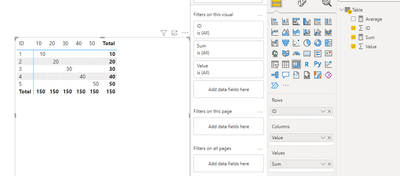Hope this helps you. Here is my PBIX file.

Best Regards,

Community Support Team _Yinliw

If this post helps, then please consider Accept it as the solution to help the other members find it more quickly.